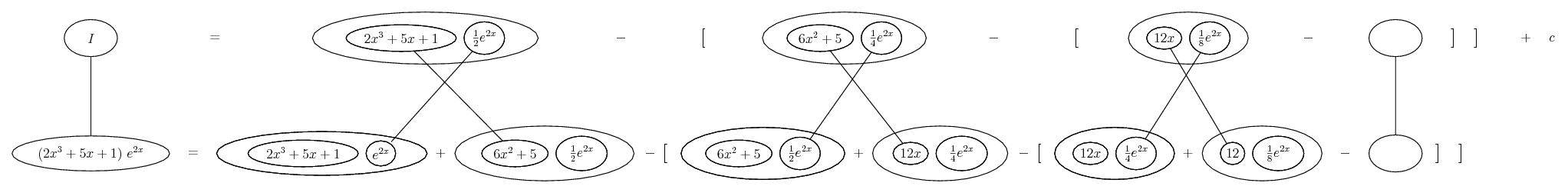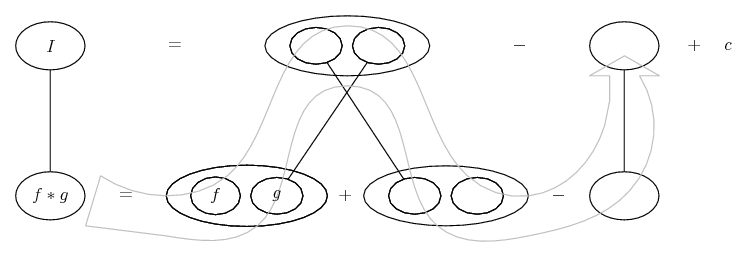# Thread: basic integration help needed

1. ## basic integration help needed

Hello guys, just finish learning about Derivatives and Limits yesterday, so I thought I could get started on Integration now.

I've looked in alot of places but can't find out how to integrade a multiplication/division! I mean, addition and subtraction are easy stuff, just distribute the integral sign but how are multiplication, division handled?

Here:
Integrate: 3x(Cos(x^3 + 7))
Integrate: (x+1)/(x-1)

Thanks for any help!
Derg

2. Dear Dergyll,

In the first integration have you written,$\displaystyle \int{3xCos(x^3+7)dx}\mbox{ or }\int{3Cos(x^3+7)dx}$ Please confirm this and then I will try to help.

3. Sorry about that,

your first integration is correct, its a 3 "x" not multiplication.

4. Dear Dergyll

2)$\displaystyle \int{{\frac{x+1}{x-1}}dx}=\int{\frac{x-1+2}{x-1}}dx=\int{dx}+2ln\mid{x-1}\mid+C=x+2ln\mid{x-1}\mid+C$

I shall try to do your first sum when time permits. But for the moment the answer escapes me.

5. $\displaystyle \int{3xCos(x^3+7)dx}$ cann't be expressed as elementary function, see Wolfram.

6. Ok, but how do I use the integration by parts method? Why if the problem occurs something like this?

$\displaystyle \int (2x^3+5x+1) (e^2x) dx$

7. Just in case a picture helps...... bigger...

Spoiler:... where... is the product rule. Straight continuous lines differentiate downwards (integrate up) with respect to x. And integration by parts is a matter of filling out this rule usefully but starting (unlike in differentiation) with one of the lower corners instead of at the top. So the basic pattern is...... and choosing legs crossed or uncrossed is equivalent to asigning u, v, and du and dv

By the way, other brands of IBP are available. E.g. tabular. And of course this is 'integration by parts thrice', because we have to repeat the pattern until the order of the polynomial goes to zero.

And I take it this is a new example you're asking about - if you wanted to tackle the 3x(Cos(x^3 + 7)) by parts then, no, no deal. Can't be expressed as elementary means can't be done, basically (roughly).

__________________________________________

Don't integrate - balloontegrate!

Balloon Calculus; standard integrals, derivatives and methods

Balloon Calculus Drawing with LaTeX and Asymptote!

8.Originally Posted by DergyllIntegrate: (x+1)/(x-1)
Derg
Try u = x-1
then (x+1)/(x-1) = ? (in terms of u)
dx = ?

#### Search Tags

basic, integration, needed